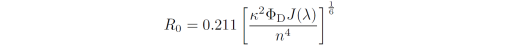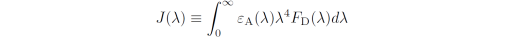### The Spectral Overlap Integral

In fluorescence resonance energy transfer (FRET) the donor-acceptor distance at which the FRET efficiency is 50%, R0, is a function of the spectral overlap between the donor fluorescence spectrum and the acceptor absorption spectrum. If the wavelength is in nm then the critical distance is in Ångström given bywhere k is an orientation factor between the donor and acceptor, n is the refractive index, FD is the fluorescence quantum yield of the donor in absence of the acceptor and J is the overlap integral between the normalized emission spectrum of the donor and the absorption spectrum of the acceptor. Here J is in units of M-1cm-1nm4 and is defined bywhere eA is the extinction coefficient spectrum of the acceptor in units of M-1cm-1l  is the wavelength in nm and FD is the wavelength dependent donor emission spectrum normalized to an area of 1.

### Calculating spectral overlap integrals in a|e

The a|e freeware provides a one-click shortcut to calculate multiple spectral overlap integrals, independent of the measured wavelength data grids of the individual spectra. In this example the dye spectra were imported directly into a|e from an online database. Alternatively, you can also load your own spectra into a|e.

1. Make sure the acceptor absorption spectra are in units of M-1cm-1, i.e. the extinction coefficient spectra.

If this is the case go directly to step 2.

If this is not the case, use the 'Add / multiply spectrum by a constant' function to make the extinction coefficient spectra:

1a. First activate the 'Calculator' toggle button in the toolbar. This will activate the 'Add / multiply spectrum' panel.

1b. Use the datacursor tool to select the wavelength at which you know the extinction coefficient. In this case we select the FAM absorption spectrum at it's peak, 492 nm, and see that the value is currently A = 100.

1c. Knowing that the peak extinction coefficient should be e ~78,000 M-1cm-1 a value of 780 is entered into the edit box followed by pressing the 'times' (*) button. The spectrum is now multiplied by a value of 780 resulting in the desired extinction coefficient spectrum. The FAM spectrum is used as an example here - FAM is of course the donor in this particular case.

1d. Redo step 1b-c on all other acceptor absorption spectra. In this case the peak extinction coefficient of TAMRA is e ~91.000 M-1cm-1 and the peak extinction coefficient of Alexa610 is e ~138.000 M-1cm-1.

2. To calculate the spectral overlap integrals between all absorption and emission spectra plotted in the graph window, press the 'Calculate spectral overlap integral' button in the toolbar.

The result is shown in the message board. Note that a|e automatically normalizes all emission spectra before calculating J (behind the scene), so this step is not needed manually by the user.